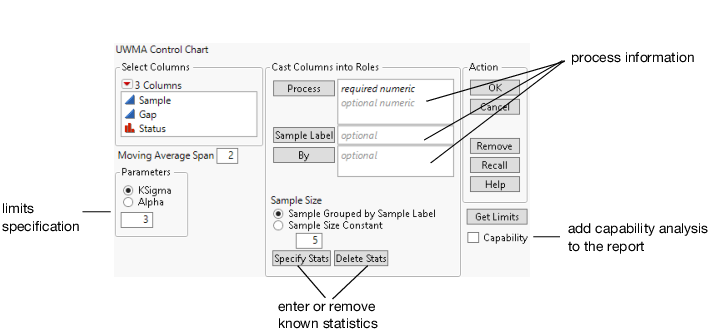Publication date: 08/13/2020

## Statistical Details for Average Run Length

The one-sided average run length (ARL) values are calculated using the integral equation method (with 24 Gaussian points) described by Goel and Wu (1971). If the Head Start value is greater than 0, the values are calculated according to the method in Appendix A.1 of Lucas and Crosier (1982).

Note that the two-sided ARL values are related to the positive and negative one-sided ARL values by the following equation:Lucas and Crosier (1982) describe the properties of a Head Start value for CUSUM charts in which the initial CUSUM S0 is set to a nonzero value. This is sometimes referred to as a fast initial response (FIR) feature. Average run length calculations given by them show that the FIR feature has little effect when the process is in control and that it leads to a faster response to an initial out-of-control condition than a standard CUSUM chart.